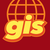# Calculate polygon area and perimeter in geodesic

730
3
03-04-2021 10:10 PMOccasional Contributor

Hi,

After adding polygon I want to calculate area and perimeter in geodesic unit. I'm able to calculate area and convert it into hectares but not sure how to calculate perimeter in kilometer.

Area I'm using below code.

``````var ft = (Feature)row;
if (ft.GetShape().GeometryType == GeometryType.Polygon)
{
var area = GeometryEngine.Instance.GeodesicArea(ft.GetShape()) / 10000;
//Calculate and convert area from meter to hectares
}``````

I couldn't find function to calculate perimeter in GeometryEngine.

Any help would be appreciated.

-Prashant

1 Solution

Accepted SolutionsOccasional Contributor III

Did you try creating a polyline from segments (see example here) and passing that to GeometryEngine.GeodesicLength ?

3 RepliesbyEsri Regular Contributor

Currently there is no option to add unit in the GeometryEngine, mostly are meters by default.

How I solved for that situation at the moment is - divided by 10 ^ 6 or (1000 * 1000) to get square meters.

Similarly for Nautical Miles divided by 1852 ^ 2 or (1862 * 1852) for area value.

Best Regards,

ThanOccasional Contributor III

Did you try creating a polyline from segments (see example here) and passing that to GeometryEngine.GeodesicLength ?Occasional Contributor

Hi,

Thanks for the help. Working fine. Here is my sample code.

``````ICollection<Segment> collection = new List<Segment>();
poly.GetAllSegments(ref collection);
double lenght = 0;
foreach (var item in collection)
{
var polyline = new PolylineBuilder(item);
lenght += GeometryEngine.Instance.GeodesicLength(polyline.ToGeometry()) / 1000;
}``````